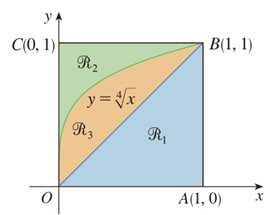Chapter 5.2, Problem 21E

Chapter
Section
Textbook Problem

# Refer to the figure and find the volume generated by rotating the given region about the specified line.ℛ 1 about AB

To determine

To find:

The volume generated by rotating the region R1 about the line  AB by using the figure.

Explanation

1) Concept:

i. If the cross section is a disc and the radius of the disc is in terms of x  or y then the area is A=π radius2

ii. The volume of the solid revolution about the y-axis is

V= abA(y)dy

2) Given:

Figure:

3) Calculation:

Here, OB appears to be the line y=x and AB appears to be the line x=1.

Rotating R1 about AB that is the line  x=1, the cross section is perpendicular to the y-axis and it is a disc with the radius r=1-y because it is the distance from the line OB (y=x)  to the axis of the rotation line AB (x=1).

From the figure, the solid lies between y=0 and  y=1

### Still sussing out bartleby?

Check out a sample textbook solution.

See a sample solution

#### The Solution to Your Study Problems

Bartleby provides explanations to thousands of textbook problems written by our experts, many with advanced degrees!

Get Started

#### Simplify: 810

Elementary Technical Mathematics

#### True or False: If f(x) 0 for all x in an interval I then f is increasing on I.

Study Guide for Stewart's Single Variable Calculus: Early Transcendentals, 8th

#### Show that elnx=x.

College Algebra (MindTap Course List)